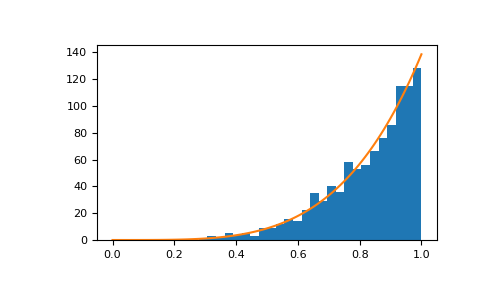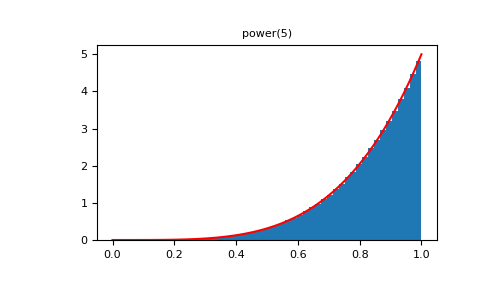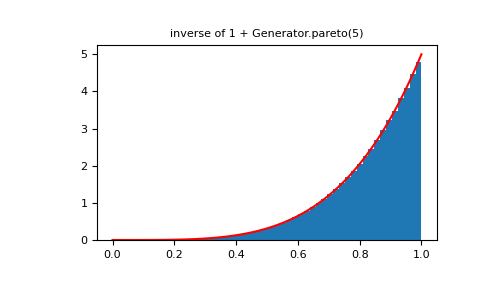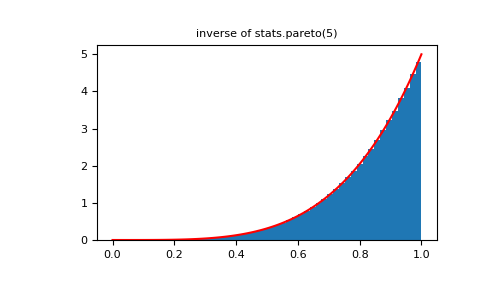#### Previous topic

numpy.random.Generator.poisson

#### Next topic

numpy.random.Generator.rayleigh

# numpy.random.Generator.power¶

method

`Generator.``power`(a, size=None)

Draws samples in [0, 1] from a power distribution with positive exponent a - 1.

Also known as the power function distribution.

Parameters
afloat or array_like of floats

Parameter of the distribution. Must be non-negative.

sizeint or tuple of ints, optional

Output shape. If the given shape is, e.g., `(m, n, k)`, then `m * n * k` samples are drawn. If size is `None` (default), a single value is returned if `a` is a scalar. Otherwise, `np.array(a).size` samples are drawn.

Returns
outndarray or scalar

Drawn samples from the parameterized power distribution.

Raises
ValueError

If a < 1.

Notes

The probability density function isThe power function distribution is just the inverse of the Pareto distribution. It may also be seen as a special case of the Beta distribution.

It is used, for example, in modeling the over-reporting of insurance claims.

References

1

Christian Kleiber, Samuel Kotz, “Statistical size distributions in economics and actuarial sciences”, Wiley, 2003.

2

Heckert, N. A. and Filliben, James J. “NIST Handbook 148: Dataplot Reference Manual, Volume 2: Let Subcommands and Library Functions”, National Institute of Standards and Technology Handbook Series, June 2003. https://www.itl.nist.gov/div898/software/dataplot/refman2/auxillar/powpdf.pdf

Examples

Draw samples from the distribution:

```>>> rng = np.random.default_rng()
>>> a = 5. # shape
>>> samples = 1000
>>> s = rng.power(a, samples)
```

Display the histogram of the samples, along with the probability density function:

```>>> import matplotlib.pyplot as plt
>>> count, bins, ignored = plt.hist(s, bins=30)
>>> x = np.linspace(0, 1, 100)
>>> y = a*x**(a-1.)
>>> normed_y = samples*np.diff(bins)*y
>>> plt.plot(x, normed_y)
>>> plt.show()
```Compare the power function distribution to the inverse of the Pareto.

```>>> from scipy import stats
>>> rvs = rng.power(5, 1000000)
>>> rvsp = rng.pareto(5, 1000000)
>>> xx = np.linspace(0,1,100)
>>> powpdf = stats.powerlaw.pdf(xx,5)
```
```>>> plt.figure()
>>> plt.hist(rvs, bins=50, density=True)
>>> plt.plot(xx,powpdf,'r-')
>>> plt.title('power(5)')
```
```>>> plt.figure()
>>> plt.hist(1./(1.+rvsp), bins=50, density=True)
>>> plt.plot(xx,powpdf,'r-')
>>> plt.title('inverse of 1 + Generator.pareto(5)')
```
```>>> plt.figure()
>>> plt.hist(1./(1.+rvsp), bins=50, density=True)
>>> plt.plot(xx,powpdf,'r-')
>>> plt.title('inverse of stats.pareto(5)')
```